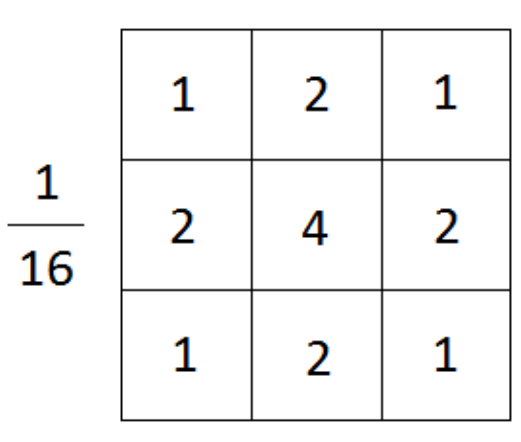# How to apply the Lorentzian function to simulated images?

I found that using aBSDF significantly overestimated DGP values because I did not applied the blurring algorithm/filter mentioned in Greg’s Paper and Jan’s presentation.

I wonder how we could apply the Lorentzian function to hdr images by Gaussian function with FWHM=11. Any suggestion is appreciated, many thanks!

Best,
Sichen

Here is a c-shell script you can use to apply the blur. Save the code as a .csh file (e.g. `blur.csh`) and simply run on command line: `blur.csh test.hdr > blurred.hdr`

``````#!/bin/csh -fe
#
# Simulate Lorentzian function with FWHM=11
#
getinfo < \$1 | sed 's/^EXPOSURE=.*\$/EXPOSURE=1.0/' > myhead\$\$.txt
set vhoriz=`sed -n 's/^VIEW=.*-vh \([^ ]*\) .*\$/\1/p' myhead\$\$.txt`
echo \$vhoriz
set rhoriz=`getinfo -d < \$1 | sed 's/^.*+X //'`
set pparcmin=`ev "\$rhoriz/(60*\$vhoriz)"`
if ( "\$rad" !~ [1-9]* ) then
echo "Input image is too small for this script"
exit 1
endif
if ( "\$rad" !~ [1-9]* ) then
pcomb -s .85 -o \$1 -s .11 -o "\!pgblur -r \$rad \$1" \
-s .04 -o "\!pgblur -r \$rad \$1" \
| getinfo -
else
pgblur -r \$rad \$1 | pcomb -s .85 -o - -s .11 -o "\!pgblur -r \$rad \$1" \
-s .04 -o "\!pgblur -r \$rad \$1" \
| getinfo -
endif
``````

Hi Taoning,

Thank you so much for sharing the code. It seems like this csh file can only be ran in a unix-like system, but I am not familiar with those systems. I am trying to understand the code and the algorithm in this paper, so I could come up with a code for Windows.

My question is how to define the size and standard deviation of a Gaussian kernel based on the given information such as spatial frequency (e.g.: 10 cycles per degree), radii, or “11% given to 2.6 cycles/degree”. For example, a 3x3 Gaussian kernel with a standard deviation of 1, will look like this (image source):Many thanks,
Sichen

Here we’re assuming equi-angular projection, and once we figure out pixel/arcmin for our image we can apply the standard deviation from the paper as pixel radius (-r) to pfilt. Lorentian with FHWM=11 roughly mapped to standard deviation of 5.7 for gaussian. We(Greg) also added two additional blur at larger radius and weighted them at end to have a better fit to the lorentian (I guess?)

In Radiance, the actual blurring is done in pfilt. Here I have the equivalent Python script, maybe you can try the it on windows: `python blur.py test.hdr out.hdr`

``````import argparse
import math
import os
import subprocess as sp
import sys
import tempfile

if reduc <= 1:
return sp.run(["pfilt", "-1", "-r", str(radii), inp], check=True, stdout=sp.PIPE).stdout
dims = sp.run(['getinfo', '-d'], input=inp.encode()).stdout.split()
pfilt1 = sp.run(['pfilt', '-1', '-x', f"/{reduc}", '-y', f"/{reduc}", inp], check=True, stdout=sp.PIPE)
pfilt2 = sp.run(['pfilt', '-1', '-r', str(filt), '-x', dims, '-y', dims], input=pfilt1.stdout, check=True, stdout=sp.PIPE)
return pfilt2.stdout

def humanblur(inp):
"""Simulate Lorentzian function with FWHM=11"""
header = sp.run(['getinfo', inp], check=True, stdout=sp.PIPE).stdout
view_line = [l for l in header.decode().splitlines() if l.strip().startswith('VIEW=')]
parser = argparse.ArgumentParser()
args, _ = parser.parse_known_args(view_line.split())
vhoriz = args.vh
out = sp.run(['getinfo', '-d', inp], check=True, stdout=sp.PIPE).stdout.decode().split()
rhoriz  = int(out)
pparcmin = rhoriz / (60 * vhoriz)
rad = 5.7 * pparcmin, 22.6 * pparcmin, 42.4 * pparcmin
raise ValueError("Input image is too small")
with tempfile.TemporaryDirectory() as td:
blur1 = os.path.join(td, "blur1")
blur2 = os.path.join(td, "blur2")
with open(blur1, 'wb') as tmp:
with open(blur2, 'wb') as tmp2:
proc = sp.run(['pcomb', '-s', '.85', '-o', inp, '-s', '.11', '-o', blur1, '-s', '.04', '-o', blur2],
check=True, stdout=sp.PIPE)
return proc.stdout
else:
with tempfile.TemporaryDirectory() as td:
blur1 = os.path.join(td, "blur1")
blur2 = os.path.join(td, "blur2")
with open(blur1, 'wb') as tmp:
with open(blur2, 'wb') as tmp2:
proc = sp.run(['pcomb', '-s', '.85', '-o', '-', '-s', '.11', '-o', blur1, '-s', '.04', '-o', blur2],
check=True, input=blur0, stdout=sp.PIPE)
return proc.stdout

if __name__ == "__main__":
with open(sys.argv, 'wb') as out:
out.write(humanblur(sys.argv))

``````

Hi Taoning,

Thank you for explaining algorithm and sharing the code. It works well and blurs the image.

I deleted this line of code, but it is not working on my system.

dims = sp.run([‘getinfo’, ‘-d’], input=inp.encode()).stdout.split()

Instead, I manually entered the image size (1000 by 1000) to the code.

pfilt2 = sp.run([‘pfilt’, ‘-1’, ‘-r’, str(filt), ‘-x’, ‘1000’, ‘-y’,‘1000’], input=pfilt1.stdout, check=True, stdout=sp.PIPE)

Best,
Sichen

It should probably be

``````dims = sp.run([‘getinfo’, ‘-d’], input=inp.encode(), stdout=sp.PIPE).stdout.split()
``````